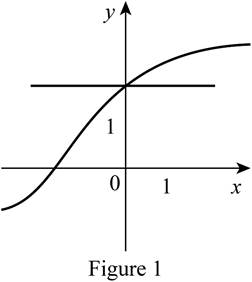# Why the given graph f is one-to-one.### Single Variable Calculus: Concepts...

4th Edition
James Stewart
Publisher: Cengage Learning
ISBN: 9781337687805### Single Variable Calculus: Concepts...

4th Edition
James Stewart
Publisher: Cengage Learning
ISBN: 9781337687805

#### Solutions

Chapter 1.6, Problem 18E

(a)

To determine

## To explain: Why the given graph f is one-to-one.

Expert Solution

### Explanation of Solution

Perform the horizontal line test for the given graph.

Draw a horizontal line such that it passes through the curve as shown in Figure 1.From Figure 1, it is observed that the horizontal line intersects the curve exactly at once which means it passes the horizontal line test. Therefore, the function is an one-to-one function.

(b)

To determine

### To find: The domain and range of f−1 .

Expert Solution

The domain of f1 is [−1, 3].

The range of f1 is [−3, 3].

### Explanation of Solution

Notice that the domain of the graph f is [−3, 3] and the range of the graph f is [−1, 3].

According to the definition of an inverse function, the domain of f1 is the range of f and the range of f1 is the domain of f.

Thus, the domain of f1 is [−1, 3] and the range of f1 is [−3, 3].

(c)

To determine

### To find: The value of f−1(2) .

Expert Solution

The value of f1(2)=0 .

### Explanation of Solution

From part (a) it is known that the function is one-to-one.

It is identified from the graph that f(0)=2 .

According to the definition of an inverse function, f1(y)=x if and only if f(x)=y where f is one-to-one.

The value of f1(2)=0 since f(0)=2 .

Thus, it can be concluded that the value of f1(2)=0 .

(d)

To determine

### To estimate: The value of f−1(0) .

Expert Solution

The value of f1(0)1.7 .

### Explanation of Solution

From part (a) it is known that the function is one-to-one.

It is identified from the graph that f(1.7)=0 .

According to the definition of an inverse function, f1(y)=x if and only if f(x)=y where f is one-to-one.

The value of f1(0)1.7 since f(1.7)=0 .

Thus, it can be estimated that the value of f1(0)1.7 .

### Have a homework question?

Subscribe to bartleby learn! Ask subject matter experts 30 homework questions each month. Plus, you’ll have access to millions of step-by-step textbook answers!